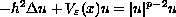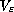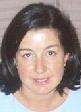Electron. J. Diff. Eqns., Vol. 2001(2001), No. 09, pp. 1-14.

### Multiple solutions to some singular nonlinear Schrodinger equations Monica Lazzo

Abstract:
We consider the equationwhich arises in the study of standing waves of a nonlinear Schrodinger equation. We allow the potentialto be unbounded below and prove existence and multiplicity results for positive solutions.

Submitted October 3, 2000. Published January 22, 2001.
Math Subject Classifications: 35Q55, 35J20, 58E05.
Key Words: nonlinear Schrodinger equations, singular potentials, variational methods, Ljusternik--Schnirelman category.

Show me the PDF file (168K), TEX file, and other files for this article.Monica Lazzo Dipartimento di Matematica Universita di Bari via Orabona 4, 70125 Bari, Italy e-mail: lazzo@dm.uniba.it